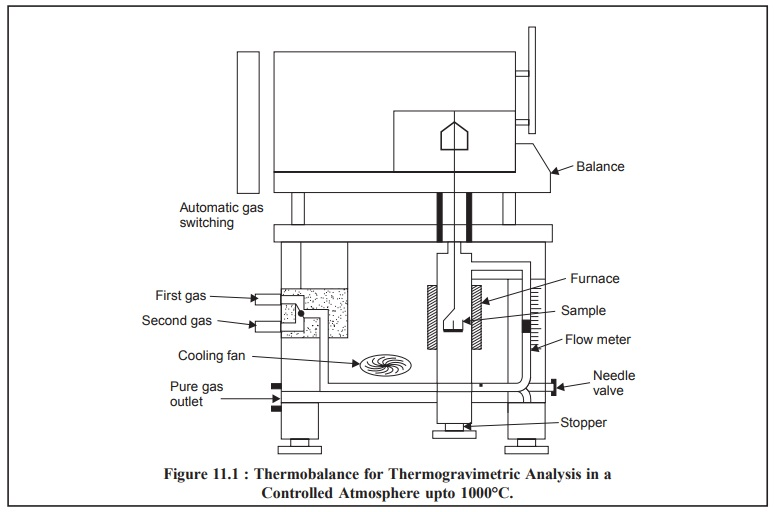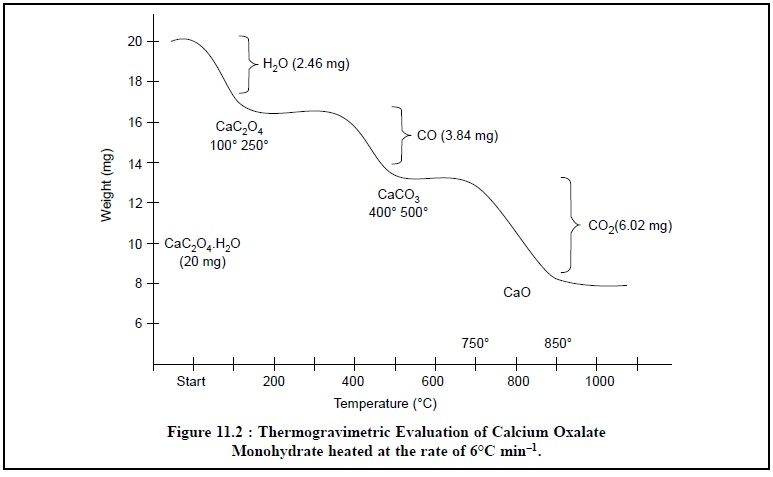Home | | Pharmaceutical Drug Analysis | Thermogravimetric Analysis (TGA)

# Thermogravimetric Analysis (TGA)A large number of chemical substances invariably decompose upon heating, and this idea of heating a sample to observe weight changes is the underlying principle of thermogravimetric analysis (TGA).

THERMOGRAVIMETRIC ANALYSIS (TGA)

## 1. THEORY

A large number of chemical substances invariably decompose upon heating, and this idea of heating a sample to observe weight changes is the underlying principle of thermogravimetric analysis (TGA). However, TGA may be sub-divided into two heads, namely :

(a) Static (or Isothermal) Thermogravimetric Analysis, and

(b) Dynamic Thermogravimetric Analysis.

### 1.1. Static Thermogravimetric Analysis

In this particular instance the sample under analysis is maintained at a constant temperature for a period of time during which any changes in weight are observed carefully.

### 1.2. Dynamic Thermogravimetric Analysis

In dynamic thermogravimetric analysis a sample is subjected to conditions of predetermined, carefully controlled continuous increase in temperature that is invariably found to be linear with time.

## 2. INSTRUMENTATION

The essential requirements for an instrument (Figure 11.1) meant for thermogravimetric analysis are, namely :

(a) A high-precision balance,

(b) A furnace adequately programmed for a linear rise of temperature with time, and

(a)       A sensitive recorder.### 2.1. Balances

They are usually of two types :

(a) Null-point Type : It makes use of an appropriate sensing-element which aptly detects any slightest deviation of the balance beam and provides the application of a restoring force, directly propor-tional to the change in weight, thereby returning the beam to its original null-point. The restoring-force is subsequently recorded either directly or with the aid of a transducer.

(b) Deflection Type : It is essentially based on either a conventional analytical balance consisting of helical spring, cantilever beam and strain gauze or a torsion analytical balance involving the conversion of deviations directly into a record of the weight change.

### 2.2. Furnace

The furnace must be designed in such a fashion so as to incorporate an appropriate smooth input thereby maintaining either a fixed temperature or a predetermined linear-heating programme (e.g., 10°C-600°C per hour).

The temperature control of the furnace is satisfactorily achieved via a thermocouple mounted very close to the furnace-winding. The maximum operational temperature may be obtained by using different thermocouples as indicated below :### 2.3. Recorder

The recording device must be such so as to :

(i) record both temperature and weight continuously, and

(ii) make a definite periodic record of the time.

## 3. METHODOLOGY

The ‘thermogram’ for calcium oxalate monohydrate (CaC2O4.H2O) is presented in Figure 11.2. The successive plateaus correspond to the anhydrous oxalate (100-250°C), calcium carbonate (400-500°C), and finally calcium oxide (700-850°C). In other words, these plateaus on the decomposition curve designate two vital aspects, namely :

(a) clear indication of constant weight, and

(b) stable phases within a specified temperature interval. The chemical reactions involved may be summarized as follows :### 3.1. Interpretation of Thermogram

In  the  thermogram  (Figure  11.2),  which  vividly  illustrates  the  thermogravimetric  evaluation  of CaC2O4.H2O, it is ensured that the weight of this product decreases in several stages, namely :Stage 1 : The water of hydration (or crystallization) from calcium oxalate monohydrate is lost which corresponds to 2.46 mg (12.3%) equivalent to 1 mole of H2O in the temperature range 100-250°C.

Actually, the 12.3% weight loss that took place between 100-250°C should correspond to 12.3% of the original formula weight for CaCO3 H2O (FW = 146). Hence, the product being lost has a formula weight of 0.123 × 146 = 17.958 ( ~ 18.0), and it corresponds to H2O.

Stage 2 : One mole of carbon monoxide is evolved subsequently from calcium oxalate, corresponding to 3.84 mg (19.2%) in the temperature range 400-500°C.

The 19.2% weight loss that occurred between 400-500°C should correspond to 19.2% of the original formula weight of 146. Therefore, the product being given out has a formula weight of 1.92 × 146 = 28.0, that corresponds to CO.

Stage 3 : Finally, a mole of CO2 is evolved from calcium carbonate that corresponds to 6.02 mg (3.01%) in the temperature range 700-850°C.

The weight loss amounting to 3.01% which took place in the range 700-850°C must, in fact, corresponds to 3.01% of the original formula weight of 146. Therefore, the product being evolved has a formula weight of 0.301 × 146 = 43.946 ( ~ 44), and it corresponds to CO2.It is quite evident that in a multicomponent system wherein more than one component exhibits weight variations and that too at different temperature regions, the composition of the original compound may be estimated as depicted in Figure 11.2.

In a situation whereby an inert material is present along with a pure substance, from the generated thermogram the composition of the mixture may be derived from the percentage weight variation which takes place relative to the percentage weight variation observed with the pure compound (A), by employing the following expression :## 4. APPLICATIONS

The most broadly based application of the thermogravimetric analysis (TGA) has been visualized and exploited in the investigation of analytical methods, such as :

(i) Determining appropriate forms for many elements,

(ii) Screening and testing of substances which may be used as potential analytical standards (primary standard), and

(iii) Direct application of the technique in analytical assays.

A few typical applications of TGA are, namely :

(a) Plateaus for hydrates are sometimes based on the initial water content (i.e., water of crystalliza-tion). It has been observed that in humidified air at low heating rates, hydrates usually give rise to good plateaus.

Example : Dehydration of sodium tungstate 28-hydrate [Na2WO4.28 H2O (5 : 12)]

Experimental parameters* :

A. Humidified air, 300°C/hour,

B. Humidified air, 150°C/hour,

C. Humidified air 10°C/hour,

D. Room air, 10°C/hour,

Sample weight : 0.5000 g ;

n = Moles water per 5Na2O, 12 WO3

(b) Analysis of flue-gas scrubber system in environmental analysis.

The flue-gas emitted from a coal-fired-power-plant is subjected to scrubbing by the aid of wet limestone to get rid of sulphur dioxide (SO2) as completely as possible. TGA helps in monitoring the system by carrying out the analysis of the products resulting from the scrubbing process, that mainly consist of (i) CaCO3 ; (ii) CaSO3 . CaSO3 . 1/4 H2O, and (iii) CaSO4 . 2H2O.

The thermogram obtained from TGA provides the following valuable informations which suggests the decomposition occurring at three distinct stages thereby causing the loss due to two moles of water, half-a-mole of water and one mole of CO2.(c) The stepwise degradation of organic polymers has received adequate attention which has broadened the in-depth knowledge of polymer chemistry. In this specific instance the sample is either heated under vacuum or in an inert atmosphere (of N2).

(d) The thermogravimetric data may be employed to evaluate the kinetic parameters of weight varia-tions in reactions.

Study Material, Lecturing Notes, Assignment, Reference, Wiki description explanation, brief detail
Pharmaceutical Drug Analysis: Thermoanalytical Analysis : Thermogravimetric Analysis (TGA) |

Related Topics